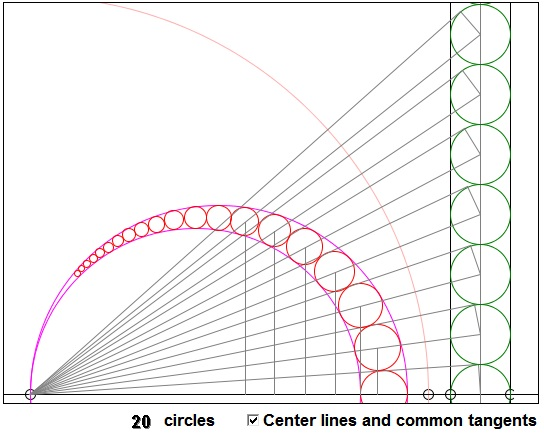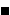# Chain of Inscribed Circles

Construct two tangent circles Σ1 and Σ2 and the line L through their centers. We are going to inscribe into the crescent-shaped space between the circles a chain of pairwise tangent circles. The first one, α0, has its diameter on L. The second, α1, is tangent to α0, and both Σ1 and Σ2, and so on. Let hn and Rn denote the distance to L from the center and the radius of the circle αn. Then

 (1) hn = 2n·Rn.

### If you are reading this, your browser is not set to run Java applets. Try IE11 or Safari and declare the site https://www.cut-the-knot.org as trusted in the Java setup.What if applet does not run?

Indeed, an inversion with the center at the common point of tangency of Σ1 and Σ2 maps the two circles onto two parallel lines perpendicular to L. The circles αn map an a chain of equal circles inscribed between the two parallel lines with the diameter of the first one on L. For those circles, the analogue of (1) is obvious: h'n = 2n·R'n, where h'n is the distance from the center of the inverse image of αn to L and R'n is its radius. However, the circles in every inverse pair are homothetic with the center of homothety at the center of inversion. Let dn and d'n be the distances from the center of inversion to the centers of circle αn and its inverse image. Then comparing the similar triangles formed by their line of centers and the common tangent gives a proportion

dn/Rn = d'n/R'n.

Now looking ad the second pair of similar triangles, viz., those formed by the center line and the horizontal axis we get

hn/dn = h'n/d'n.

Whence, hn/Rn = h'n/R'n = 2n.(The above proposition is commonly referred to as Pappus Circle Theorem or Pappus Chain Theorem. Pappus was of course unaware of the inversion. His proof is much more elaborate. He himself refers to it as "The Ancient Theorem" [Gaba].)

We can easily derive an additional result.

Assume the radius of the right semicircle is k times the radius of the left one. If the former is RR, and the latter is RL, we assume that

 (2) RR = k·RL.

Let R denote the radius of the big semicircle, then, since R = RR + RL, (2) implies that

 (3) RL = R/(1 + k) and RR = R·k/(1 + k).

For the sake of simplicity, let's choose the radius of the circle of inversion to be 2R. Then the left vertical line (this is the one that corresponds to the big semicircle) becomes tangent to both the big and the right semicircles as the common point of tangency lies then on the circle of inversion and is thus fixed. The right vertical line corresponds to the left semicricles. Introduce further notations. Let On be the center of an and Pn the projection of On on L. Let AB, CB, and AC denote the diameters of the semicircles. A is the center of inversion, AB = 2R, AC = 2RL, CB = 2RR, C' is the inversion of C, O' is the midpoint of BC'.(2R)² = AB² = AC·AC'.

Thus

AC' = (2R)²/AC = 4R²/2RL = 2(1 + k)R.

And, since AO' = (AB + AC')/2,

AO' = (2 + k)R,
BO' = kR.

So, for the same reason as (1),

hn/APn = 2nBO'/AO', or
Rn/APn = BO'/AO'

And

 (4) APn = AO'·Rn/BO' = (2 + k)Rn/k.

Next, let's derive a generalization of Archimedes' formula:

 (5) Rn = Rk / (k²n² + k + 1).

The points of tangency of the common tangents through A of a pair of corresponding circles (like Tn and T'n in the diagram) play a double role. On one hand, they are homologuous, corresponding under the implied homothety. On the other hand, they are antihomologous, mapping on each other under the inversion. More accurately

AT'n / ATn = R'n / Rn = Rk / Rn, and also
AT'n · ATn = (2R)²

implying

(AT'n)² = 4R3k / Rn.

But (AT'n)² = (2R + kR)² + (2n·kR)² - (kR)² which gives an equation for Rn, with the solution

Rn = Rk / (n²k² + k + 1).

which is (5). The combination of (4) and (5) gives

 (6) APn = R(k + 2) / (k²n² + k + 1).

Note that, t = 1/k, so that RL = t·RR then (5) becomes

 (5') Rn = Rt / (t² + t + n²).

### References

1. M. G. Gaba, On a Generalization of the Arbelos, Am Math Monthly, Vol. 47, No. 1 (Jan., 1940), 19-24### Inversion - Introduction